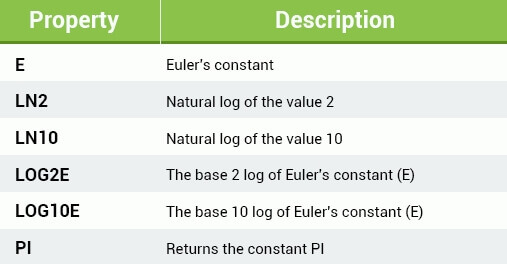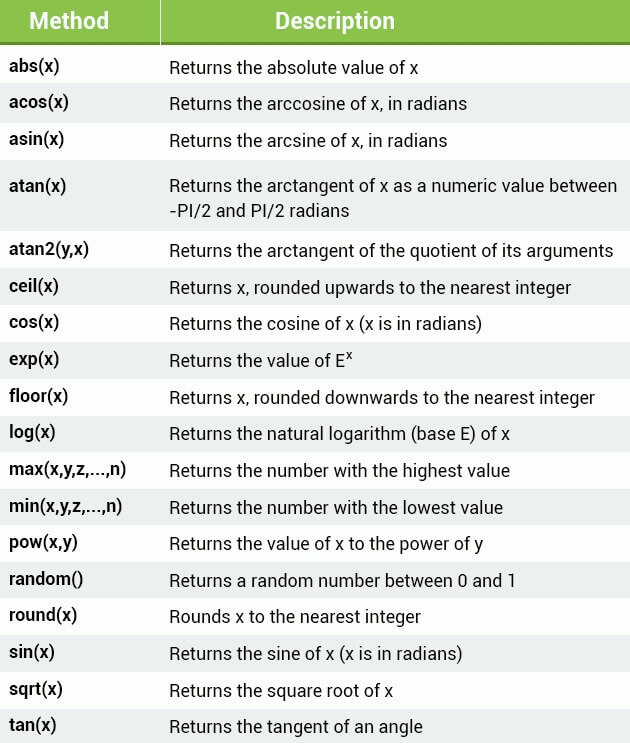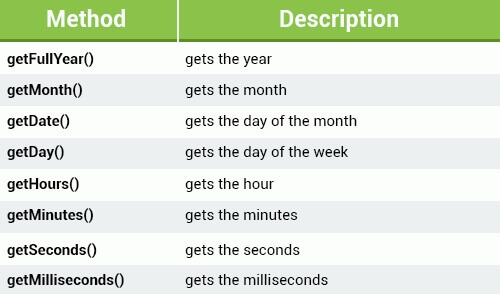# JavaScript 入门教程之六 -- JS内置 Objects

### JavaScript Arrays 数组

``````var course1 ="HTML";
var course2 ="CSS";
var course3 ="JS"; ``````

``var courses = new Array("HTML", "CSS", "JS");``

``````var courses = new Array("HTML", "CSS", "JS");
var course = courses;
courses = "c++";``````

``````var courses = new Array(3);
courses = "HTML";
courses = "CSS";
courses = "JS";``````

array 数组是一种特殊的 object，它使用 index number 来访问元素，而标准 object 使用 property name 来访问元素，name 是字符形式。

``````var arr = {
0: 0,
1: 1,
2: 2,
3: 3
}

var obj = {
'0': 0,
'1': 1,
'2': 2,
'3': 3
}``````

JavaScript array 是动态的，也就是你可以在创建时不传入任何参数给构造器：

``````var courses = new Array();
courses = "HTML";
courses = "CSS";
courses = "JS";
courses = "C++";``````

``var courses = ["HTML", "CSS", "JS"];``

JavaScript array 内建了很多实用的 properties 和 method。

length property 返回数组的元素个数：

``````var courses = ["HTML", "CSS", "JS"];
document.write(courses.length);``````

`length` 的返回值比最后一个元素的索引号大 1，如果数组为空，则返回值为 0。

concat method 可以叠加两个数组并返回为一个新的数组：

``````var c1 = ["HTML", "CSS", "JS"];
var c2 = ["C++"];
var newC = c1.concat(c2);``````

newC 数组拥有四个元素："HTML", "CSS", "JS", "C++"。注意使用 `concat()` method 并不会影响 c1 和 c2。

`map` method 可以给数组每个元素调用一个 function 然后返回修改后的数据为一个新数组：

``````var arr = [1, 2, 3, 4];
var newArr = arr.map(function(i) {
return i * 2;
})
console.log(newArr);

//output:
//0: 2
//1: 4
//2: 6
//3: 8
//length: 4``````

`reduce` Method 可以将数组元素合并为一个 value。语法为：

``````array.reduce(function(total, currentValue))
``````

total 为元素合并的累积值，currentValue 为当前循环处理到的元素。调用 reduce 时，第一次传入 total 为 第一个元素数据，currentValue 为数组第二个元素，function 会返回一个结果作为第二次循环 total 的数据，currentValue 指向第三个元素，然后进行循环调用 function 累积 total 的值，最后返回 total。

``````var arr = [1, 3 ,5];
var sum = arr.reduce(function(total, curr) {
});
console.log(sum);

//output:
//9``````

`filter` method 可以逐个过滤数组的元素，返回符合条件元素创建一个新数组。语法如下：

``````array.filter(function(currentValue))
``````

filter 将数组元素逐个作为传入参数调用 function，function 返回值为 Boolean 类型，如果为 true 则返回当前数组元素，反之则丢弃当前数组元素。

``````var arr = [1, 4, 6, 3, 8];
var newArr = arr.filter(function(i) {
return i < 5;
});

console.log(newArr);

//OUTPUT:
//0: 1
//1: 4
//2: 3
//length: 3``````

`join` method 将数组所有元素转换为一个字符串并返回这个字符串。下面是一个示例：

``````var arr = ['a', 'b', 'c'];
var x = arr.join();
console.log(x);

//output:
//a,b,c``````

``````var arr = ['a', 'b', 'c'];
var x = arr.join('/');
console.log(x);

//output:
//a/b/c``````

`indexOf` method 搜索一个数组中特定的元素，并返回这个元素所在 index 索引。语法如下：

``````array.indexOf(item, start)
``````

item 是搜索的元素，start 定义开始搜索的位置，默认为 0。

``````var arr = ['a', 'b', 'c', 'a', 'e', 'b'];
var x = arr.indexOf('b');
var y = arr.indexOf('b', 2);
var z = arr.indexOf('f');
console.log(x);
console.log(y);
console.log(z);

//output:
//1
//5
//-1``````

Associative Arrays 关联型数组

``````var a = [];
a["name"] = "marco";
a["age"] = 20;
document.write(a["age"]);``````

JavaScript 会将 a 作为 object 处理，这样 name 和 age 就是其 properties。可以使用以上写法来读取 property 数据。

JavaScript 原生并不支持命名化的元素索引，所以推荐当你想要使用 number 数字型 index 时使用 array，当你想要使用命名化的索引时使用 object。

### the Math Object 数学对象

the Math Object 可以用来处理数学运算任务，它包含多个 properties：Math 没有 constructor 构造器，所以使用时并不需要单独创建 object。例如：

``````document.write(Math.PI);
``````

Math object 包含多个 method 用来计算：``var a = Math.sqrt(4);``

``````Math.ceil(Math.random() * 10);
``````

``````var n = prompt("please input a number: ", "");
var m = Math.sqrt(n);

### The Date Object 时间对象

`setInterval()` method 用来在指定的间隔时间(毫秒)下调用 function 或评估一个表达式。它将会持续调用直到执行 ` clearInterval()` 或关闭窗口。

``````function myAlert() {
}

`Data` object 可以让我们使用时间元素，一个 `Date` object 由：a year, a month, a day, an hour, a minute, a second, and milliseconds 构成。

``````var d = new Date();
//d stores the current date and time``````

``````new Date(milliseconds)
new Date(dateString)
new Date(year, month, day, hours, minutes, seconds, milliseconds)``````

JavaScript 日期计算使用毫秒为单位，起始日期为：01 January, 1970 00:00:00 (UTC)。一天包含 86,400,000 毫秒。

``````//Fri Jan 02 1970 00:00:00
var d1 = new Date(86400000);

//Fri Jan 02 2015 10:42:00
var d2 = new Date("January 2, 2015 10:42:00");

//Sat Jun 11 1988 11:42:00
var d3 = new Date(88,5,11,11,42,0,0);``````

JavaScript 的月份从 0 到 11，1 月就是 0，12 月就是 11。Date object 是 static 类型的，创建后就不会改变。

Date object 有如下 method 可供使用：``````var d = new Date();
document.write(d.getHours());``````

``````function printTime() {
var d = new Date();
var hour = d.getHours();
var min = d.getMinutes();
var sec = d.getSeconds();
document.body.innerHTML = hour + ":" + min + ":" + sec;
}

setInterval(printTime, 1000);``````

`innerHTML` property 可以设置或返回一个 HTML 元素的内容，这里我们将 document 的 body 块的内容赋值为我们定义的时间数据，并且每秒覆盖更新一次。